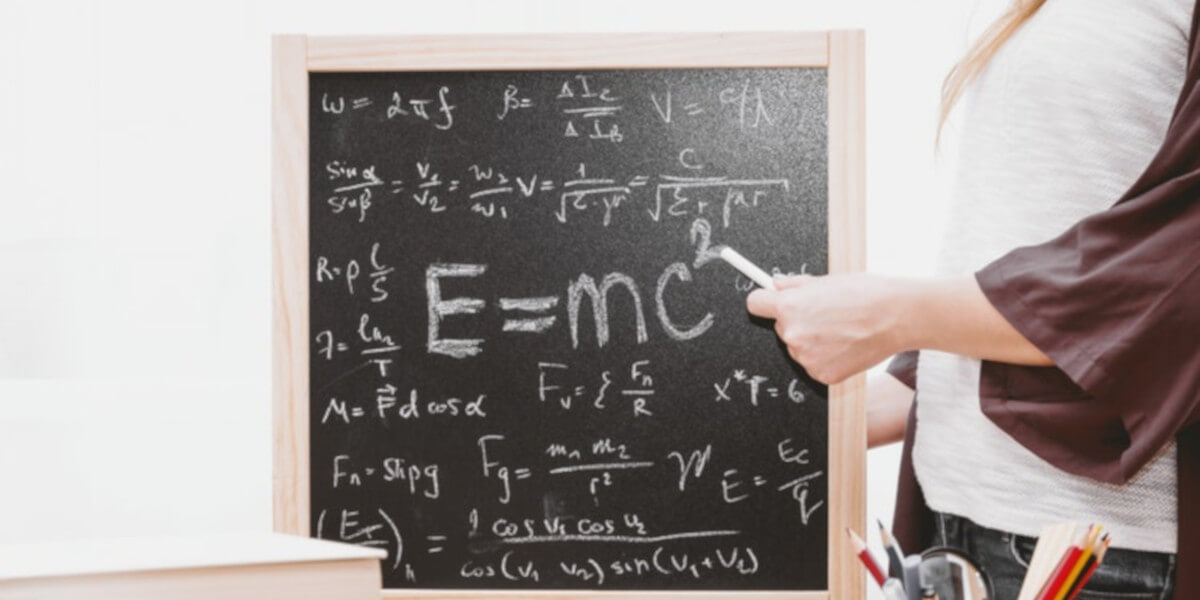# Bachelors (BSc) in Applied Mathematics Abroad

## Overview

Applied Mathematics is a professional discipline wherein the core mathematical methods are applied by different fields such as physics, engineering, medicine, biology, business, computer science, etc. Developing solutions to new problems from different fields requires a sound knowledge of various branches of mathematics, such as analysis, differential equations and stochastics, utilizing analytical and numerical methods. So, emphasis of the discipline is both on the mathematical methods and practical problems which are to be solved.

The aim of the process is to develop new or improved methods to solve the real-world problems that arise in diverse areas. Thus, Applied Mathematics can be termed as a combination of mathematical science with specialized knowledge.

Do you have an ardent interest in mathematics with a knack for problem solving? A Bachelor’s program in Applied Mathematics can be a worthy consideration for your undergraduate studies, as it will not only help you develop a robust understanding of different branches of mathematics but also apply the same to real world problems.

What differentiates this course from regular mathematics is that it entails practical application of mathematical theories. It also creates a platform for students to combine their interest in mathematical reasoning, with other interests in a specific field of intellectual activity or research.

## Course Structure

Major course topics taught under the Applied Mathematics program deal with practical applications and problem resolution in varied disciplines. Thus, course structure and topics are designed to instill the required skills among students. Below is the list of core courses in Bachelor's in Applied Mathematics

• Calculus
• Discrete Mathematics
• Introduction to Analysis
• Partial Differential Equations
• Transferable Skills for Applied Mathematicians
• Scientific Computing
• Introduction to Uncertainty Quantification

## Course Subjects

Applied Mathematics is a research intensive field of study demanding a firm grasp of the required subjects while pursuing a Bachelor's degree. Following are the subjects required for Bachelor’s in Applied Mathematics

• Abstract Algebra
• Differential Equations
• Applied Probability
• Discrete Mathematics for Computer Science
• Physics
• Problem-solving and Proof-writing
• Linear Algebra
• Number Theory
• Combinatorics

## Key skills :

• Number Sense. This is the ability to count accurately—first forward.
• Representation.
• Spatial sense.
• Measurement.
• Estimation.
• Patterns.
• Problem-solving.

## Applied Mathematics scholarships 2021 - 2022

• New Zealand Aid Programme 2021-2022 Scholarships for international students
• Global Korea Scholarship - Funded by Korean Government
• Friedrich Ebert Stiftung Scholarships
• ANU Chancellor’s International Scholarship
• STEM funding - Full Sail University for international Students

## Some of the job roles after studying Applied Mathematics are

• Economist
• Statisticians
• Actuary
• Physicists and Astronomer
• Mathematician
• Market Research Analyst

## FAQs

Blogs

POPULAR COURSES

Masters

Bachelors

TOP UNIVERSITIES

By Country

By Course

YOCKET TOOLS & SERVICES